# How long are your shoelaces?

 How long are your shoelaces? The answer is, of course, “It depends”. It depends on how you lace your shoes. Searching the web, there are three very common styles of basic lacing (and dozens of fancier other methods, some involving more than one set of laces).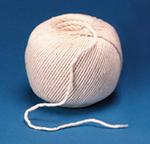The three I'm going to look at, I’m going to call Criss-Cross, Straight laced and Bow-Tie. (I’m sure there are probably official technical names for them, but these are the names that I’m going to use!)For all the patterns above I’ve used red to represent the part of the lace going “down” the shoe, and green for the path “up”. The first two lacing patterns I’m analyzing have something in common: The binding alternates eyelets left-right as they hold the shoe together.

The Bow-Tie pattern, however, sometimes runs the laces up the same side.

### If the shoe fits …

Different shoe designs have different numbers of pairs of eyelets. In this analysis, I’m using the letter n to represent the number of pairs of eyelets.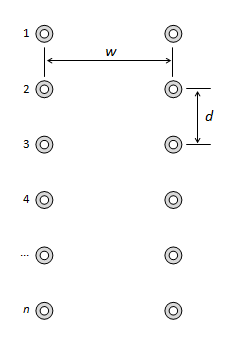We'll use the letter d to describe the distance separating each row of eyelets, and the letter w for the distance between the eyelets on each side when the shoe is pulled tight. (I’m assuming, for this analysis, that the rows are parallel and equidistant).
 Using some high-school geometry, and a little Pythagoras, it’s possible to work out the lengths of the intermediate segments of shoe lace. For instance, on the right we see the length of a simple cross-over diagonal.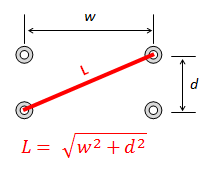For all lace calculations, the flying-ends of the laces are assumed to be constant, so we're just looking at comparing the length of the laces inside the shoe. Here are the results:

### Criss-CrossFor Criss-Cross, there is the straight section at the bottom of the shoe w, followed by (n-1) pairs of simple diagonals.

### Straight-LacedFor Straight-Laced, there are (n-1) sets of horizontal bars and simple diagonals, plus one large diagonal which returns the lace from the bottom corner to the opposite top corner.

### Bow-Tie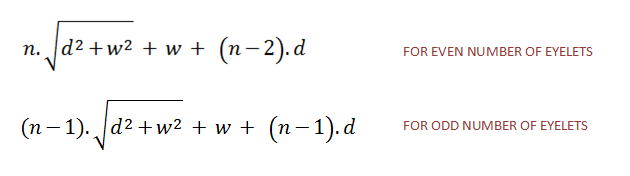For Bow-Tie, things are a little more complex because it depends whether there are an odd or even number of rows of eyelets (the pattern alternates between criss-cross and side-lacing).

With an odd number of eyelet rows, there are the same quantity of straight sections and crossed sections (plus the bottom bar). With an even number of eyelet rows, there is one more section of crossed than straights (This is assuming, like in the earlier diagram, that you install the laces with a cross section at the bottom of the design. It's possible to alternate the design pattern so that the lowest section is straight and not crossed. If you select this design, the switch the n and the (n-2) terms in the top equation).

### Plot

Plotting a chart of these three equations shows that, straight-laced always required the longest length of lace, Criss-Cross shorter, and Bow-Tie shorter still.The Bow-Tie lines has steps because of the alternation between the odd and even eyelet formulae. Also note that, when the number of rows of eyelets is two (and trivially one!), all the designs are the same, and the required lengths of the laces is correspondingly the same.

Further analysis (outside of the scope of this posting) shows that, for patterns that alternate left-right, the Criss-Cross pattern is the most efficient design. I'll try and show this graphically, even if not mathematically.

To do this, examine the plots below. These are scalar plots of the length of the laces. Each move to the right in the chart shows a measurement of the lace in the w axis of the shoe, and each move upwards in the chart shows a measurement in the d axis of the shoe. The graph is sort of like each lace unraveled and attached on a peg-board. Each lace has to traverse the graph from one corner to the diametric opposite corner (completing the number of vertical steps and horizontal steps to alternate left-right, and to move from top-bottom-top of the shoe).

The purple dots in the center of the chart represent the eyelets at the ‘bottom’ of the shoe (with the top and bottom row representing the ‘top’ of the shoe).The Criss-Cross plot is shown above. The line pretty much traces the leading diagonal (with the exception of the stair in the middle which is the bottom cross-over and is common in all lace patterns). It's not hard to imagine that a straight line leading from one corner of the plot to the other is the shortest distance between these two points, and thus the corresponding lace pattern that follows this route is the shortest method to lace up a shoe.

This shows that the Criss-Cross pattern is the most efficient lacing style that alternates left-right through a shoe.

The chart below shows scalar path of the Straight-Laced pattern.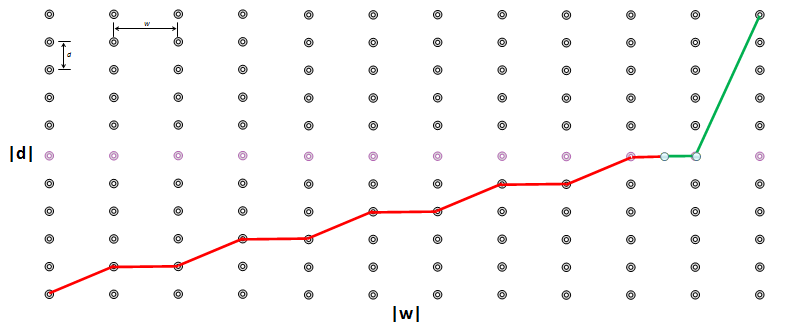You can see above how the line that connects the opposite corners is longer, and this pattern uses more lace than the Criss-Cross method.

Finally, for completeness, here is a plot of the Bow-Tie method. Here, the line does not extend all the way to the right. (All it needs to do is reach from the bottom row to the top row).Removing the constraint that the lace needs to alteranate left-right, as can be see above, allows the line in the scalar plot to be made shorter as its gradient increases. (This can be achieved by threading the lace through more eyelets on the same side and not crossing to the other side).

# Trivia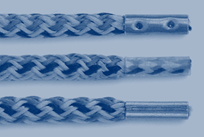A common trivia question: What do you call the piece on the end of a shoelace that stops it fraying? Answer: Aglet.

You can find a complete list of all the articles here.Click here to receive email alerts on new articles.